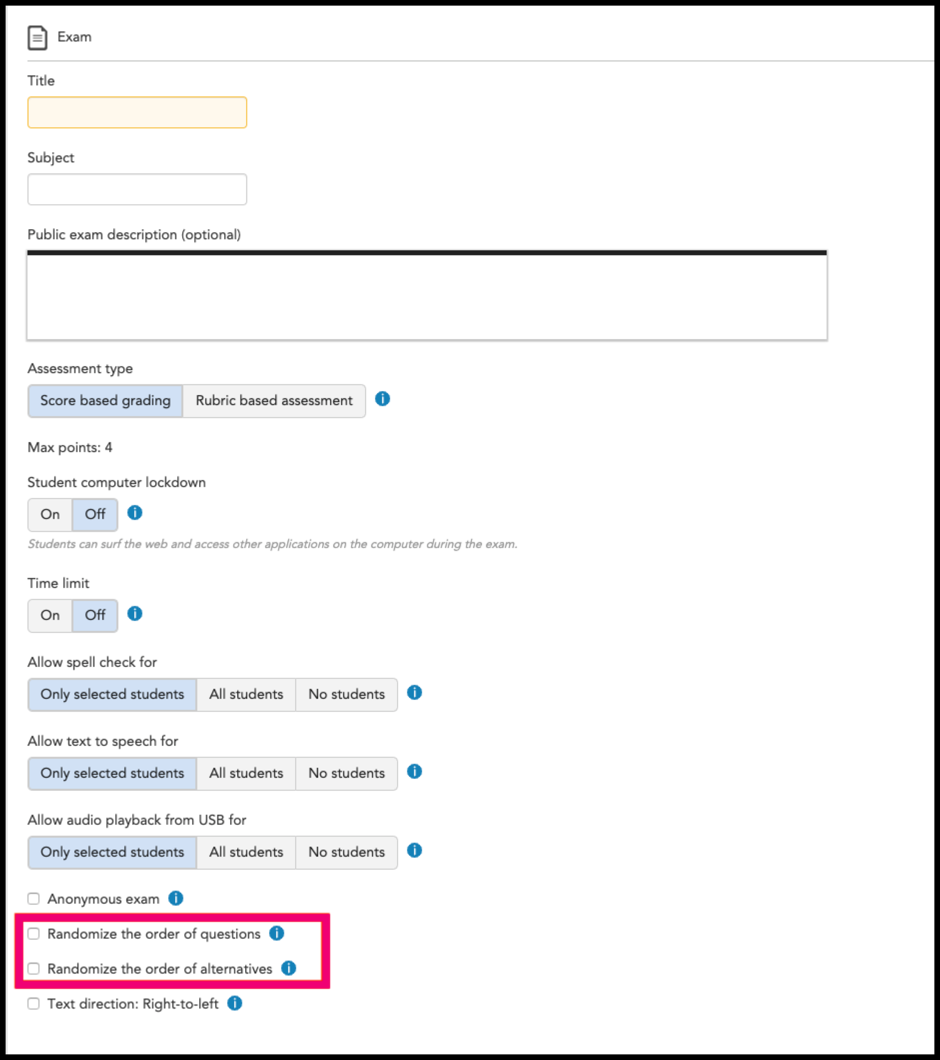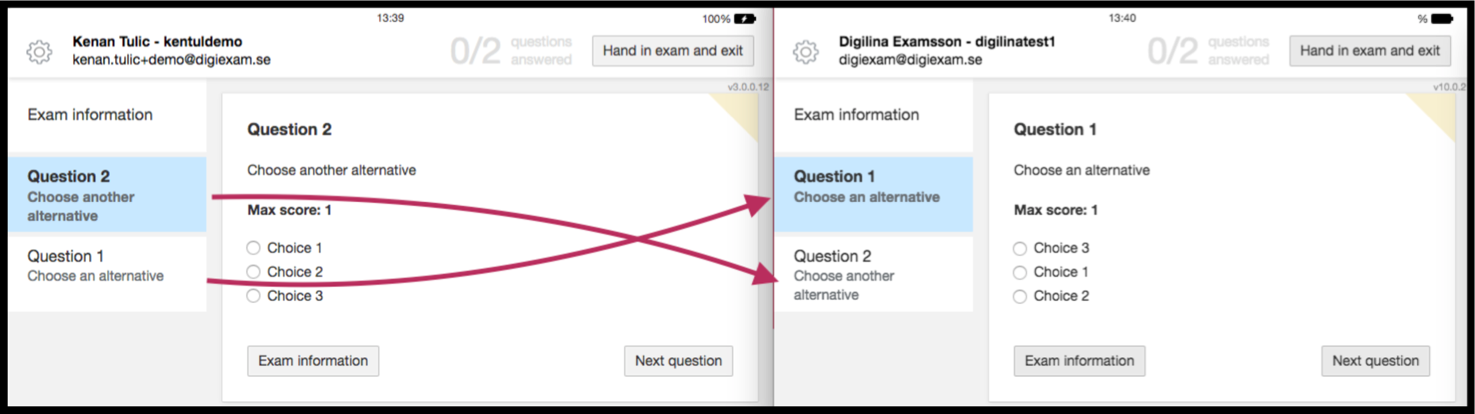Follow

# Randomization feature

When creating assessments, exam creators are able to randomize the order of questions and/or randomize the order of answer choices. In order to enable these settings, exam creators will need to create an exam or edit an existing exam.

Then exam creator should check the boxes Randomize the order of questions and/or Randomize the order of alternatives based on their preference (figure 1).Figure 1

## Randomization from a student's view

### Question randomization (figure 2)Figure 2

### Answer choice randomization (figure 3)Figure 3